# AML Logistic Regression Properties

LabVIEW 2018 Analytics and Machine Learning Toolkit Help

Edition Date: July 2018

Part Number: 377059B-01

»View Product InfoDownload Help (Windows Only)

Wire the untrained logistic regression model output of the Initialize Classification Model (LR) VI to the reference input of a standard Property Node to get an AML Logistic Regression Property Node. This Property Node has the following properties:

Property Access Data Type Description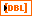Returns the trained coefficients of the trained logistic regression model. The array size equals the number of classes.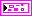Returns settings for cross validation.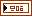If you select the Set Parameters instance of the Initialize Classification Model (LR) VI, this property returns the hyperparameters input of the VI. If you select the Search Parameters instance of the Initialize Classification Model (LR) VI, this property returns the optimal hyperparameters after the Train Classification Model VI completes grid search.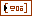Returns multiple values for each hyperparameter.Returns the method of optimization to determine the optimal hyperparameter settings.
Label of Each Class Read OnlyReturns the label of each class.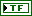Returns whether the model is initialized.